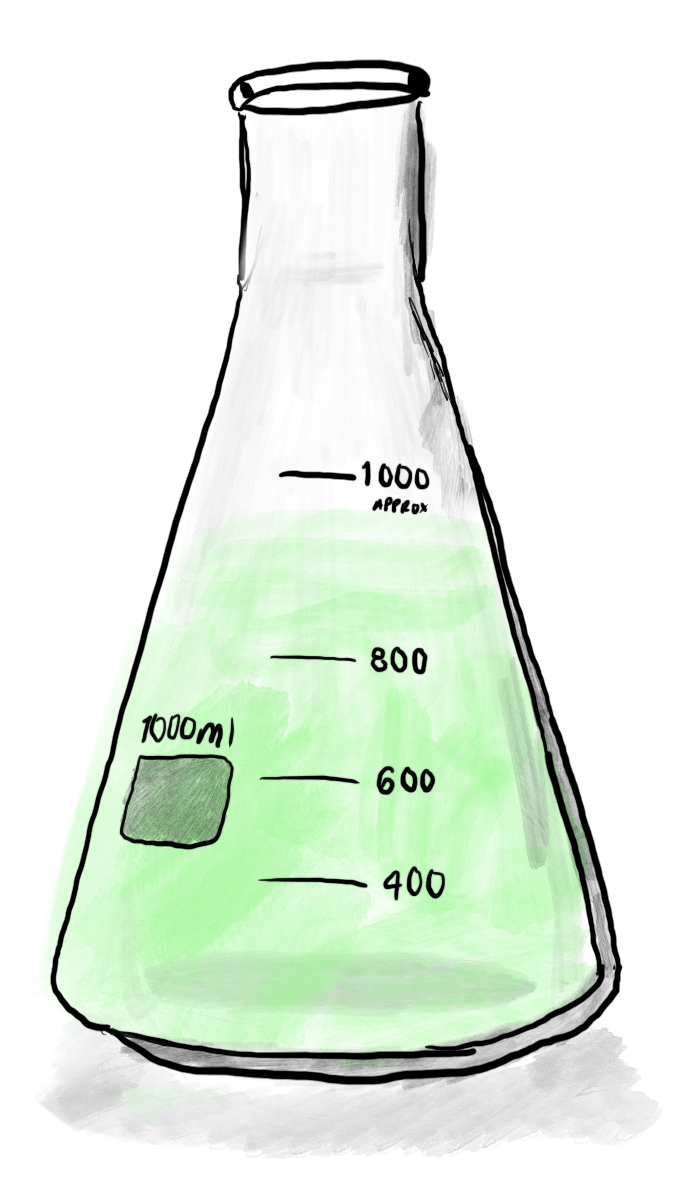# Statistical Power Analysis

## A short interactive exploration of statistical power, p-values, and experimental design.

February 6, 2019

### Experimental Design 101

Science is all about testing hypotheses. One common way that researchers do this is by running controlled experiments. While there are many different ways to do this, a common structure is to split the participants of the experiment into two groups: a control group and a treatment group.The control group acts as a baseline, serving as data points that tell how things are without any modification. The treatment group serves as the counterpoint, showing how things are with the treatment given. If you were interested in testing out a new heart medication, you would give it to members of the treatment group but not the control group, and take relevant measurements from each. This data can then be used to help assess whether or not there is a difference between the two groups. That is, did the drug work?

### P Values

In order to evaluate differences between groups, it is standard to run a statistical test that can help determine if there is a significant difference between the two groups. In order to run such a test, one first must define a null hypothesis, typically something along the lines of “there is no difference between these two groups.”

The result of a statistical test is a number, called a p-value. This number, a probability between 0 and 1, represents the likelihood that the data collected during the experiment would have been observed given that the null hypothesis there was actually no difference between the groups. If the p-value is below some predefined threshold — 0.05 is a common convention, though it varies across fields — then the null hypothesis is rejected, and your experiment serves as evidence that there is indeed a difference between the groups.

When using a p-value threshold of 0.05, there is a 5% chance of incorrectly rejecting the null hypothesis — claiming that an effect exists when none does.

The p-value can be difficult to interpret, and is notoriously easy to abuse, so it is best to treat them with care. The p-value doesn’t say anything about the size of the difference, it only tells you if it seems like there is one. If the p-value isn’t low enough to reject the null hypothesis, this isn’t evidence that the two groups are the same, rather it is only a lack of evidence that the two groups are different. Smaller differences between groups are harder to detect, and require more participants to be in the experiment in order to be identified.

### Statistical Power

The statistical power of an experiment serves as a measure of how likely an experiment is to identify a difference of a certain size between two groups. Scientists can calculate the statistical power of a particular experimental design in order to estimate how likely the experiment is to be able to identify the difference that they are hoping to see.

This calculation is important because it can tell researchers if their experiment is likely to yield any useful results, and can reveal the need for more participants to be recruited before a costly and time intensive experiment is conducted. Unfortunately, experimental power is not often reported in scientific studies, and it may not be covered in introductory statistics courses.

Similar to a p-value, the statistical power is represented as a probability between 0 and 1. It represents the probability that performing this experiment will yield a significant result, given the number of participants, the effect size, and the p-value threshold.

Adjust the numbers to update the power calculation

When conducting a comparison between two groups, given a p-value threshold of 0.05, a sample size of 50, and an effect size of 0.50 standard deviations, there is a 96% chance of seeing a significant result, given that the difference exists.

A similar calculation can be performed to find the number of experimental participants needed given a target statistical power. This is useful to understand how many participants need to be recruited for an experiment to be likely to yield useful results, and can help researchers design experiments that are resource efficient.

Adjust the numbers to update the power calculation

To conduct a comparison between two groups using a p-value threshold of 0.05, an effect size of 0.50 standard deviations, and a target power of 80%, you will need 27 participants.

Notice that the size of the difference that one is trying to identify has a large impact on the power or number of participants needed, regardless of the p-value threshold. When considering experimental design, it is important to understand the parameters, and think through their implications, rather than simply following a rote formula.

### Notes

The calculations above are for demonstrational purposes. They assume the statistical test being performed is a two-tailed t-test. These numbers will not hold for all experiments, so make sure to perform them according to the specific constraints of your experiments.

The code to calculate the power and sample size is adapted from this page. Thanks to David Schoenfeld for allowing me to use the code.

This post made with Idyll, a markdown-based language for creative interactive articles like this one. See the source code for this article here.